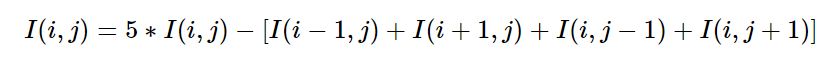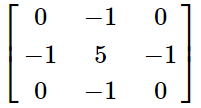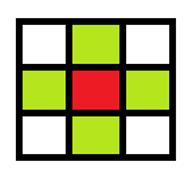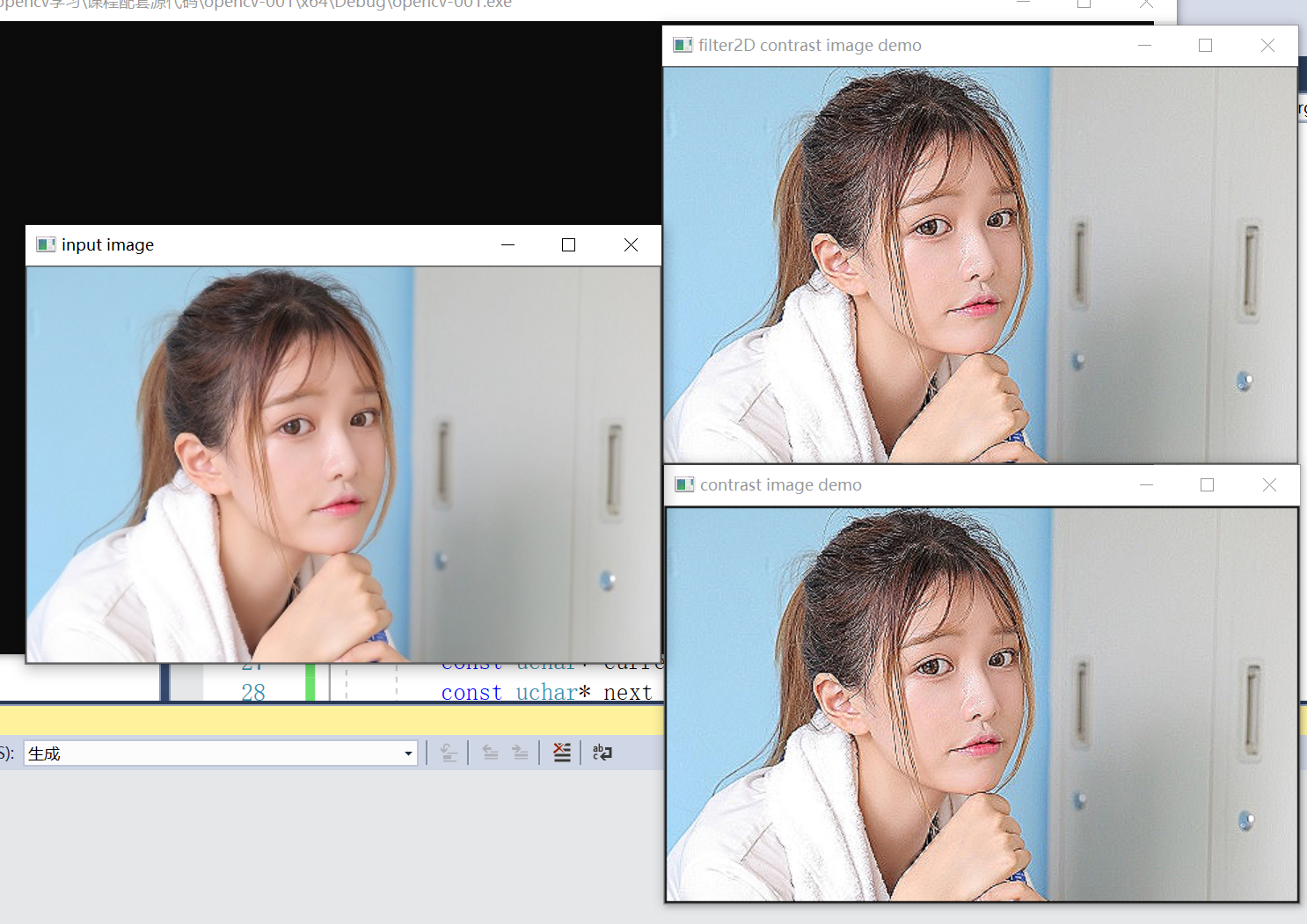WHCSRL 技术网

# OpenCV矩阵的掩码操作

## 什么是掩膜操作？### 掩膜操作实现图像对比度调整## 如何获取图像像素指针？

``````CV_Assert(myImage.depth() == CV_8U);
```1```

Mat.ptr(int i=0) 获取像素矩阵的指针，索引i表示第几行，从0开始计行数。

## 如何像素范围处理？

### 什么是像素范围处理？

``````如果我们输入小于0的值，它会返回0，

```123```

### 像素范围处理API——`saturate_cast<uchar>`

``````saturate_cast<uchar>（-100），返回 0。
saturate_cast<uchar>（288），返回255
saturate_cast<uchar>（100），返回100
```123```

## 掩膜操作的API——filter2D

``````void filter2D(
InputArray src,
OutputArray dst,
int ddepth,
InputArray kernel,
Point anchor = Point(-1,-1),
double delta = 0,
int borderType = BORDER_DEFAULT
);
```123456789```

1. `InputArray类型的src` ，输入图像。
2. `OutputArray类型的dst` ，输出图像，图像的大小、通道数和输入图像相同。
3. `int类型的ddepth`，目标图像的所需深度。
4. `InputArray类型的kernel`，卷积核（或者更确切地说是相关核）是一种单通道浮点矩阵；如果要将不同的核应用于不同的通道，请使用split将图像分割成不同的颜色平面，并分别对其进行处理。。
5. `Point类型的anchor`，表示锚点（即被平滑的那个点），注意他有默认值Point(-1,-1)。如果这个点坐标是负值的话，就表示取核的中心为锚点，所以默认值Point(-1,-1)表示这个锚点在核的中心。。
6. `double类型的delta`，在将筛选的像素存储到dst中之前添加到这些像素的可选值。说的有点专业了其实就是给所选的像素值添加一个值delta。
7. `int类型的borderType`，用于推断图像外部像素的某种边界模式。有默认值BORDER_DEFAULT。

### 使用步骤

1. 定义掩膜：`Mat kernel = (Mat_<char>(3,3) << 0, -1, 0, -1, 5, -1, 0, -1, 0);`
2. `filter2D( src, dst, src.depth(), kernel );`其中src与dst是Mat类型变量、src.depth表示位图深度，有32、24、8等。

``````filter2D( src, dst, src.depth(), kernel );
```1```

## 掩膜操作案例``````#include <iostream>
#include <math.h>
#include <opencv2/opencv.hpp>
#include<opencv2/highgui.hpp>
#include <opencv2/highgui/highgui_c.h>

using namespace cv;

int main(int argc, char** argv) {
Mat src, dst;
if (!src.data) {
printf("could not load image...
");
return -1;
}
namedWindow("input image", CV_WINDOW_AUTOSIZE);
imshow("input image", src);

// 矩阵计算 掩膜操作
int cols = (src.cols - 1) * src.channels();
int offsetx = src.channels();
int rows = src.rows;

dst = Mat::zeros(src.size(), src.type());
for (int row = 1; row < (rows - 1); row++) {
const uchar* previous = src.ptr<uchar>(row - 1);
const uchar* current = src.ptr<uchar>(row);
const uchar* next = src.ptr<uchar>(row + 1);
uchar* output = dst.ptr<uchar>(row);
for (int col = offsetx; col < cols; col++) {
output[col] = saturate_cast<uchar>(5 * current[col] - (current[col - offsetx] + current[col + offsetx] + previous[col] + next[col]));
}
}

namedWindow("contrast image demo", CV_WINDOW_AUTOSIZE);
imshow("contrast image demo", dst);

// 使用 掩膜操作API
Mat dst2;
double t = getTickCount();
Mat kernel = (Mat_<char>(3, 3) << 0, -1, 0, -1, 5, -1, 0, -1, 0);
filter2D(src, dst2, src.depth(), kernel);
double timeconsume = (getTickCount() - t) / getTickFrequency();
printf("tim consume %%%%.2f
", timeconsume);
namedWindow("filter2D contrast image demo", CV_WINDOW_AUTOSIZE);
imshow("filter2D contrast image demo", dst2);

waitKey(0);
return 0;
}
```123456789101112131415161718192021222324252627282930313233343536373839404142434445464748495051```